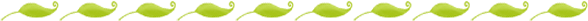# Number Sense and the Common Core: Hierarchical Inclusion, Magnitude and Subitizing

In Part 1 of this series I discussed how important number sense is to a child's development. In fact, number sense could be considered the most important part of the kindergarten year. I also discussed early number sense skills and how they relate to the Common Core. The whole of the math Common Core (except for geometry and measurement) fall under the umbrella of number sense. Here are more of the components of the Core and how they relate to an understanding of numbers.

### Hierarchal InclusionHierarchical Inclusion, as explained in the following video, is the concept that a number contains all of the previous numbers. Imagine a number as a Russian nesting doll, if working with the number 4, imagine the largest doll is 4 and inside that doll are the smaller dolls, 3, 2, 1. Without this understanding a child will think that, when counting, the number he points to and names "3" is "3" in of itself without the other objects and when you ask him for "3" he will give you only that object.

Hierarchical inclusion also helps students understand other number sense concepts, such as part/whole relationships and compensation because a child cannot understand that 4 can be broken up into the parts 3 and 1 without also understanding that the number "4" contains 3 and 1.

In order to complete the following common core standard, a child must understand hierarchical inclusion:

CCSS.MATH.CONTENT.K.CC.A.2 Count forward beginning from a given number within the known sequence (instead of having to begin at 1).

### Magnitude

Magnitude is a child's ability to compare groups. Even children who cannot count have the ability to judge the relative size of groups of objects, but as a child's number sense develops, so should the sophistication of her understanding of magnitude. The following Common Core activities depend on a child's understanding of magnitude:

CCSS.MATH.CONTENT.K.CC.B.4.C Understand that each successive number name refers to a quantity that is one larger.

CCSS.MATH.CONTENT.K.CC.C.6 Identify whether the number of objects in one group is greater than, less than, or equal to the number of objects in another group, e.g., by using matching and counting strategies.

CCSS.MATH.CONTENT.K.CC.C.7 Compare two numbers between 1 and 10 presented as written numerals.

### Subitizing

Watch the following video. In it, groups of objects quickly flash on the screen. Can you tell how many objects there are in each grouping?

As explained in the following video, subitizing is the ability of a child to quickly recognize a number visually. Children do this by mentally grouping the objects they see (and you probably did this too, for example, seeing a group of 3 and 3 and 3 and knowing that there were 9 objects).

"Looking at a quantity for a short time and then being able to tell how many are in the group(s) without counting each object in the group begins to develop from small sets of two, three, four, and five objects, to parts of sets of six up to twenty. Generally, this development begins between ages 2 and 6. Later, the subitizer sees objects as groups of 10s and 1s and, combined with an understanding of place value, is able to see the numerosity of a large group of numbers quickly." (Copley 2010).

Subitizing strengthens a child's understanding of what numbers mean, and how they relate to one another. In fact, when children are taught to subtize, and their attention is drawn to the groups and patterns they see, it becomes a visual representation of addition and subtraction, as seen here

When strengthening a child's subitizing skills, we are teaching the following Core standards:

CCSS.MATH.CONTENT.K.OA.A.1 Represent addition and subtraction with objects, fingers, mental images, drawings, sounds (e.g., claps), acting out situations, verbal explanations, expressions, or equations.

CCSS.MATH.CONTENT.K.OA.A.2 Solve addition and subtraction word problems, and add and subtract within 10, e.g., by using objects or drawings to represent the problem.

CCSS.MATH.CONTENT.K.OA.A.3 Decompose numbers less than or equal to 10 into pairs in more than one way, e.g., by using objects or drawings, and record each decomposition by a drawing or equation (e.g., 5 = 2 + 3 and 5 = 4 + 1).

CCSS.MATH.CONTENT.K.OA.A.4 For any number from 1 to 9, find the number that makes 10 when added to the given number, e.g., by using objects or drawings, and record the answer with a drawing or equation.

CCSS.MATH.CONTENT.K.OA.A.5 Fluently add and subtract within 5.

It is important to note that the word equation is present in only three of these Core standards, and in those standards it is only one option for representing addition and subtraction. In actuality, representing addition and subtraction with equations in kindergarten will not be appropriate for most of our students, but if we have the children participate in a subitizing activity where they are shown a variety of images with a quantity of 5 arranged in different ways, and a child can state that each group contains 5 because they saw a group of 1 and 4 or 2 and 3, they are fluently adding and subtracting within 5, and in a way that is more appropriate than asking them to solve 2+3=__, because instead of working from the end result backwards, we are building their foundational knowledge. In fact, testing a kindergartener's understanding of addition and subtraction by asking her to solve an equation, is like testing her phonemic awareness by asking her to read a story!

Tomorrow I will discuss the rest of the components of number sense, including the concept that the majority of your kindergarten math lessons should be focusing on. See you tomorrow!